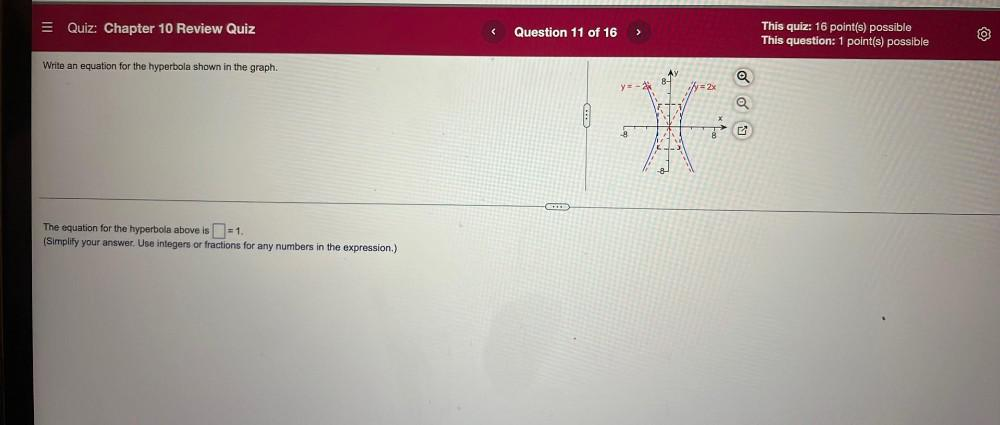Question:

# = Quiz: Chapter 10 Review Quiz < Question 11 of 16 > This quiz: 16 point(s) possible This question: 1 point(s) possible Write an= Quiz: Chapter 10 Review Quiz < Question 11 of 16 > This quiz: 16 point(s) possible This question: 1 point(s) possible Write an equation for the hyperbola shown in the graph. 8- y=-* y = 2x O -8 8 -8 The equation for the hyperbola above is (=1. . (Simplify your answer. Use integers or fractions for any numbers in the expression.)